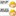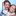Convert float to 4 Bytes and then back again

I need to convert a float to 4 bytes so it can be sent on a 8 bit data channel. I then need to convert these 4 bytes back to a float. Can anyone advice me on how to do this.

Thanks

The simplest way is just to treat it as a buffer.

float x;
...

If you need to access each byte separately, use a union:

union float2bytes { float f; char b[sizeof(float)]; };

float2bytes f2b;

float x;
f2b.f = x;
for ( int i=0; i < sizeof(float); i++ )
send_byte(f2b.b[i]);
...
for ( int i=0; i < sizeof(float); i++ )

x = f2b.f;

Note that you need to make sure the sender and receiver both use the same endianness and floating-point format.

Something like this should work. Use Google to find out more about C unions. You''ll also want to make sure you know how much C data types take up space wise.

typedef union _data {
float f;
char  s;
} myData;

#include "mbed.h"

Serial pc(USBTX, USBRX);

myData q;

int main() {
q.f = 1.234;
pc.printf("%c%c%c%c", q.s, q.s, q.s, q.s);
}

I'l leave you to work out the reverse from chars back to float :)

simplest way

float f; uint8_t *p = (uint8_t*)&f;

for (int i = 0; i < sizeof(f); i++) printf("0x%02x ", p[i]);

My solution is to employ typecasts. This makes it, of course, endianness dependent, which as explained in here, is little-endian for the LPC1768. For the examples, let's assume the float starts at position 3 of the byte array. so:

char my_array;
float my_float;

my_float = *( (float*)(my_array + 3) );           // from array to float

From float to array, any of the above solutions works as well :)

In C, the union type is often used. Something like

union floatUnion_t
{
float f;
char bytes[sizeof float]
} floatUnion1;Andy K wrote:

Something like this should work. Use Google to find out more about C unions. You''ll also want to make sure you know how much C data types take up space wise.

typedef union _data {
float f;
char  s;
} myData;

#include "mbed.h"

Serial pc(USBTX, USBRX);

myData q;

int main() {
q.f = 1.234;
pc.printf("%c%c%c%c", q.s, q.s, q.s, q.s);
}

I'l leave you to work out the reverse from chars back to float :)

Hi, im trying to convert a float variable in to hex and store it in a new variable, will this work for doing this?

Troubleshooting!

Hi guys i need some help with my code. i trying to convert readings in byte array to float with union but can't seem to work. i manage to get reading of the float but the reading is 0.00 Here is my code:

main.cpp

#include "mbed.h"

typedef union _data {
float f;
char  buffer2;
} myData;

myData q;

//--------------------------------------
//| Hyperterminal configuration         |
//| 115200 bauds, 8-bit data, no parity |
//--------------------------------------

Serial pc(SERIAL_TX, SERIAL_RX); //PC to STM32 Board
//Serial transmit(PC_4,PC_5); // STM32 writing/reading with Arduino
Serial uart(PC_4,PC_5);

//DigitalOut myled(LED1);
DigitalIn BlueButton(USER_BUTTON);  // This is Blue-Button and is on NUCLEO-L153RE
DigitalOut pc_activity(LED1);
DigitalOut uart_activity(LED2);

#define Pressed 0
#define NotPressed 1

int main() {
char buffer;
char buffer1;

//float my_float;

pc.printf("Select one of the following...");
pc.printf("\r\n");
pc.printf("i = deice type and version number");
pc.printf("\r\n");
pc.printf("r = take a single pH reading");
pc.printf("\r\n");

uart.baud(115200);                          //Setting of baud rate
int i =0;
while(1) {

uart.putc(pc.getc());

//wait(0.5);
//uart.clear();
//Sending char to arduino
pc.printf("Message Sent!\r\n");

//pc_activity = !pc_activity;       //LED indication

char tempc = uart.getc();
// if(tempc != 'v')
buffer[i] = tempc;//uart.getc();

wait(0.5);
i++;
}
}                                       //sending working
pc.printf("uart is %s",buffer);
for(int j = 0; j< 4; j++)
buffer1[j] = buffer[j+1];
pc.printf("\nnew buffer is %s",buffer1);
pc.printf("\r\n");

//my_float = *( (float*)(buffer1 + 3) );    //not working

// q.buffer2=buffer1;
//  q.buffer2=buffer1;
//  q.buffer2=buffer1;
//  q.buffer2=buffer1;

pc.printf("Float:%.2f", q.f );
}
}
}

some old functions i had laying around if anyone wants to get into bit bangin stuff you can split and or rejoin 32bits and 16bits to and from 8bits just ignore the printf's they are for testing on a local compiler, the math is in the shifts (32 bit are down a little)

////  // splits a 16bit into 2 8bits

rightshifty16 (int16_t argn)
{
unsigned char bytes;
bytes = (argn >> 8) & 0xFF;
bytes = argn & 0xFF;
printf("0x%02x 0x%02x\n", (unsigned char)bytes,
(unsigned char)bytes);
}

////  // combines 2 8bits to a 16bit

leftshifty16 (unsigned char argb[])
{
int16_t integers;
integers = (argb <<8) + argb;
printf("%d\n", (int16_t) integers);
}

////  // splits a 32bit into 4 8bits

rightshifty32 (int16_t argn)
{
unsigned char bytes;
bytes = (argn >> 24) & 0xFF;
bytes = (argn >> 16) & 0xFF;
bytes = (argn >> 8) & 0xFF;
bytes = argn & 0xFF;
printf("0x%x 0x%x 0x%x 0x%x\n", (unsigned char)bytes,
(unsigned char)bytes,
(unsigned char)bytes,
(unsigned char)bytes);
}

//// // combines 4 8bits to a 32bit

leftshifty32 (unsigned char argb[])
{
int32_t integers;
integers = (argb <<24) + (argb <<16) + (argb <<8) + argb;
printf("%d\n", (int32_t) integers);
printf("0x%x\n", (int32_t) integers);
}

Using union for byte to int32 works on x86 architecture processor but not on ARM based processor since the ARM architectures do not support sub word addressing A byte on ARM occupies a full word in memory on a ARM processor

It seems that union works also on ARM processors. Check the code below:

#include "mbed.h"

union Float {
float    m_float;
uint8_t  m_bytes[sizeof(float)];
};

float        pi;
uint8_t      bytes[sizeof(float)];
Float        myFloat;

int main()
{
pi = 3.141565f;

printf("\r\n*******************************************\r\n");
printf("*******************************************\r\n\r\n");
printf("pi = %.6f\r\n", pi);
printf("bytes = [ %d, %d, %d, %d]\r\n", bytes, bytes, bytes, bytes);

printf("\r\n***** Conversion by using type casting *****\r\n\r\n");

*(float*)(bytes) = pi;  // convert float to bytes
printf("bytes = [ 0x%.2x, 0x%.2x, 0x%.2x, 0x%.2x ]\r\n", bytes, bytes, bytes, bytes);

// send bytes over data channel ..

pi = *(float*)(bytes);  // convert bytes back to float
printf("pi = %.6f\r\n", pi);

printf("\r\n********* Conversion by using union *********\r\n\r\n");

myFloat.m_float = pi;   // assign a float to union
printf("myFloat.m_Float = %.6f\r\n", myFloat.m_float);
printf("myFloat.m_Bytes = [ 0x%.2x, 0x%.2x, 0x%.2x, 0x%.2x ]\r\n", myFloat.m_bytes, myFloat.m_bytes, myFloat.m_bytes, myFloat.m_bytes);  // get the bytes

// send bytes over the data channel ..

// receive bytes and assign them to the union bytes: myFloat.m_bytes = received_bytes ..

pi = myFloat.m_float;   // get the float back from the union
printf("pi = %.6f\r\n", pi);
}

It worksZoltan Hudak wrote:

It seems that union works also on ARM processors. Check the code below:

#include "mbed.h"

union Float {
float    m_float;
uint8_t  m_bytes[sizeof(float)];
};

float        pi;
uint8_t      bytes[sizeof(float)];
Float        myFloat;

int main()
{
pi = 3.141565f;

printf("\r\n*******************************************\r\n");
printf("*******************************************\r\n\r\n");
printf("pi = %.6f\r\n", pi);
printf("bytes = [ %d, %d, %d, %d]\r\n", bytes, bytes, bytes, bytes);

printf("\r\n***** Conversion by using type casting *****\r\n\r\n");

*(float*)(bytes) = pi;  // convert float to bytes
printf("bytes = [ 0x%.2x, 0x%.2x, 0x%.2x, 0x%.2x ]\r\n", bytes, bytes, bytes, bytes);

// send bytes over data channel ..

pi = *(float*)(bytes);  // convert bytes back to float
printf("pi = %.6f\r\n", pi);

printf("\r\n********* Conversion by using union *********\r\n\r\n");

myFloat.m_float = pi;   // assign a float to union
printf("myFloat.m_Float = %.6f\r\n", myFloat.m_float);
printf("myFloat.m_Bytes = [ 0x%.2x, 0x%.2x, 0x%.2x, 0x%.2x ]\r\n", myFloat.m_bytes, myFloat.m_bytes, myFloat.m_bytes, myFloat.m_bytes);  // get the bytes

// send bytes over the data channel ..

// receive bytes and assign them to the union bytes: myFloat.m_bytes = received_bytes ..

pi = myFloat.m_float;   // get the float back from the union
printf("pi = %.6f\r\n", pi);
}